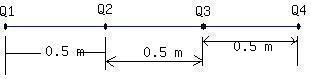Question# Four identical charges (+3.0 \mu C each)are brought from infinity and fixed to a straight line. The chargesare located 0.50 m apart. Determinethe electric potential energy of this group.

Other
ANSWEREDFour identical charges (+3.0 $$\displaystyle\mu{C}$$ each)are brought from infinity and fixed to a straight line. The chargesare located 0.50 m apart. Determinethe electric potential energy of this group.2020-12-29Initially there is no charge in the space. Whenthe charge $$\displaystyle{Q}_{{1}}$$ is brought from infinity to a point inspace, the potential energy is zero.
Secondly when the charge $$\displaystyle{Q}_{{2}}$$ is broughtfrom infinity and placed at a distance say $$\displaystyle{r}_{{{12}}}$$
from thecharge $$\displaystyle{Q}_{{1}}$$, the P.E. of the system is $$E_1=\frac{Q_1Q_2}{4\pi\epsilon_or_{12}^2}$$
$$\displaystyle{\frac{{{\left({3}\times{10}^{{-{6}}}\right)}^{{2}}\times{9}\times{10}^{{9}}}}{{{\left({0.5}\right)}^{{2}}}}}={0.324}\ {J}$$
When the third charge is brought to a point which is at a distance 0.5 m from the charge $$\displaystyle{Q}_{{2}}$$ and 1.0m from the charge $$\displaystyle{Q}_{{2}}$$ and 1.0 m from $$\displaystyle{Q}_{{1}}$$, this has to be taken in a potential; due to the above two charges. Hence the potential energy of $$\displaystyle{Q}_{{3}}$$ due to these two charges is
$$E_2=Q_3[\frac{Q_1}{4\pi\epsilon_0r_{13}^2}+\frac{Q_2}{4\pi\epsilon_or_{23}^2}]=\frac{(3\times10^{-6})^2\times9\times10^9}{0.5^2}+\frac{(3\times10^{-6})\times9\times10^9}{1.0^2}$$
So the total potential energy, $$\displaystyle={E}_{{1}}+{E}_{{2}}+{E}_{{3}}={1.17}\ {J}$$